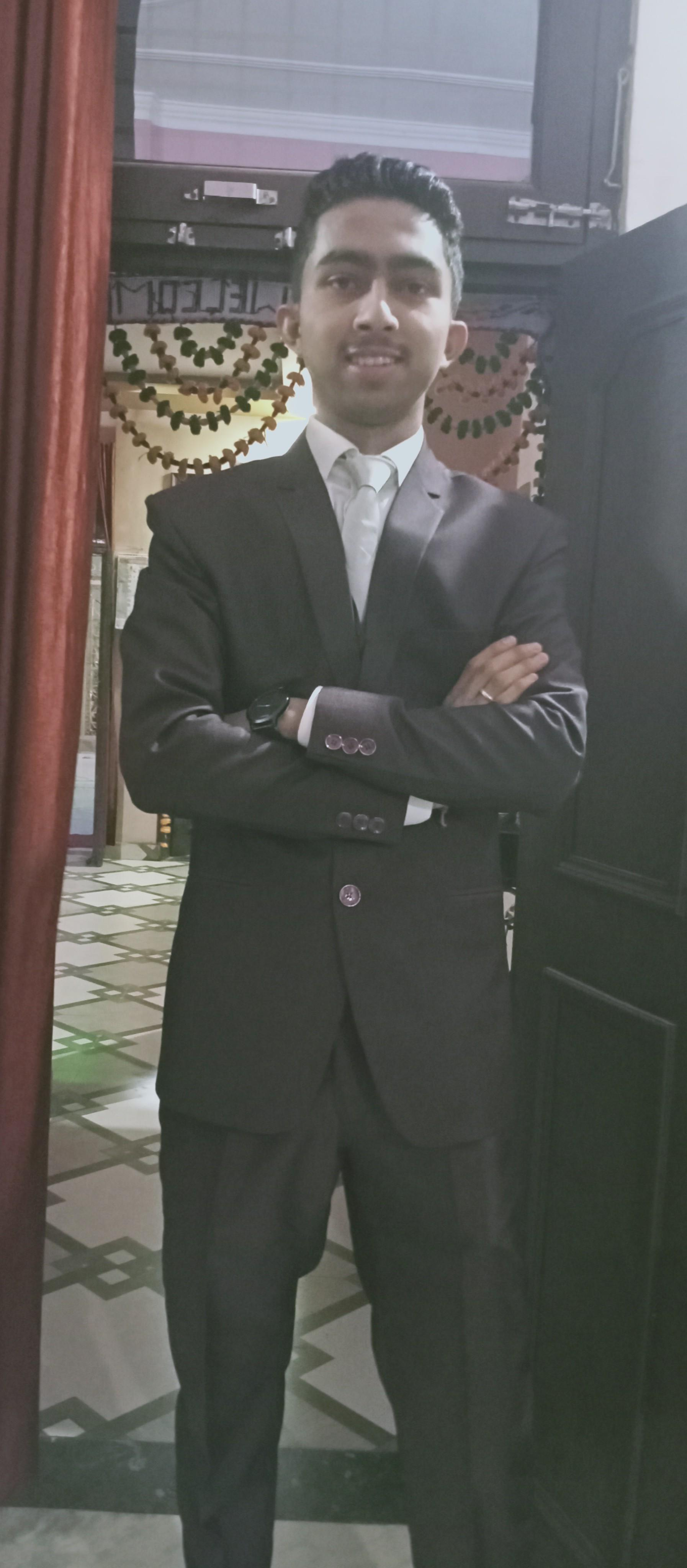• Want FREE Counselling for Exam Preparation?
###### Call Us
800-355-2211

Speak With a Friendly Mentor.###### Vijay posted an Question
July 19, 2022 • 14:44 pm 30 points
• CSIR NET
• Mathematical Sciences

## Q.51 fort e r, let [t] denote the greatest integer less than or equal to t. let d {(xy) e r2 :x2 + y2 < 4}. let f: d - r and g: d r be defined by f(0,0) = g(0,

Q.51 Fort E R, let [t] denote the greatest integer less than or equal to t. Let D {(xy) e R2 :x2 + y2 < 4}. Let f: D - R and g: D R be defined by f(0,0) = g(0, 0) = 0 and x*y2 SCx.y)= [x +ylp4yi gxy) = "+y for (x, y) # (0,0). Let E be the set of points of D at which both f and g are discontinuous. The number of elements in the set E is

• 0 Likes